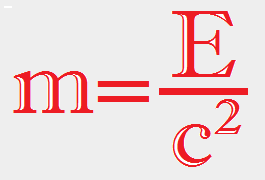Energy To Mass Calculator
If you are looking for the mass to energy calculator in which E=mc² click here.The formula shown above m = E ÷ c² has nowhere near the popularity of the legendary E=mc². Actually, (as you no doubt have already surmised), this formula is just an algebraic manipulation of E=mc². And what is the purpose of this equation and this calculator? The simplest answer is that it allows you to calculate how much energy is required to produce a certain amount of mass. Another more practical example is its use in particle physics. An electron for example, has a mass of 9.11 x 10-31 kilograms. Although accurate, that certainly is an awkward way of stating an electron's mass so, in particle physics, masses are stated in what would be their energy equaivalent. So, using E=mc², the "mass" of an electron is 511,040 electron volts or .511 Mega electron volts / c² (To make things a bit more readable, physicists usually leave out the c²)

 Finally, we can say that the purpose of this calculator is to calculate the mass of a particle when it is stated as its energy equivalent. Example 1 The Higgs Boson, an important component in theoretical particle physics, has been predicted in theory but has yet to be discovered. Whenever the Higgs Boson is mentioned in the news its mass is almost always reported as 126 GeV/c². (126 giga electronvolts or 126 billion electronvolts). What is its mass in grams? Enter 126 in the input box, click GeV, and your answer is: 2.2462 x 10-22 grams. Example 2 The Sun produces 3.828 x 1026 watts of power every second. How much mass must be converted to energy to produce this much power? Enter 3.828e26 in the input box, click joules, and your answer is: 4.2592 x 109 kilograms which is about 4,735,000 tons of mass per second!

Energy=

Significant Figures >>>
Numbers are displayed in scientific notation in the amount of significant figures you specify. For easier readability, numbers between 1,000 and -1,000 will not be in scientific notation but will still have the same precision.
You may change the number of significant figures displayed by changing the number in the box above.
Most browsers, will display the answers properly but if you are seeing no answers at all, enter a zero in the box above, which will eliminate all formatting but at least you will see the answers.